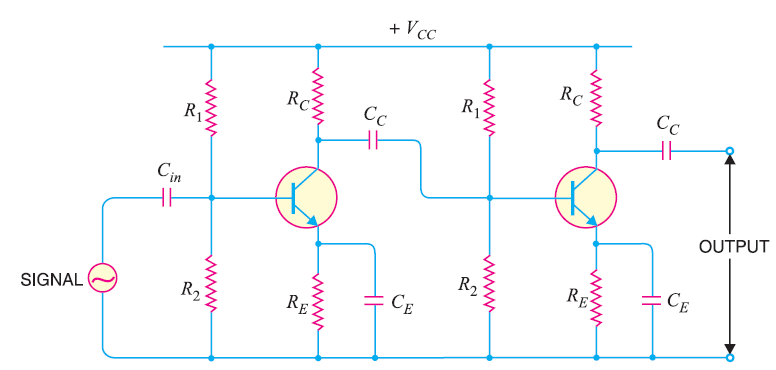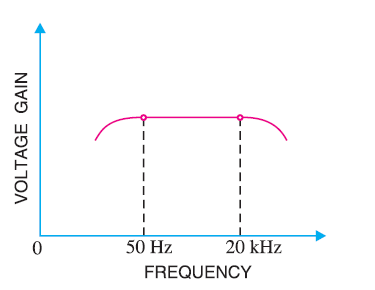# Explain Working of RC Coupled Amplifier

## RC Coupled Amplifier

Due to its low cost and excellent audio fidelity over a wide range of frequencies, an RC Coupled Amplifier is the most popular type of coupling used in a multi stage amplifier.

It is usually used for voltage amplification.

The figure below shows two stages of an RC coupled amplifier.As you can see in the fig above, a coupling capacitor Cis used to connect the output of first stage to the base i.e. input of the second stage and this continues when more stages are connected.

Since here the coupling from one stage to next is achieved by a coupling capacitor followed by a connection to a shunt resistor, therefore, such amplifiers are known as resistance-capacitance coupled amplifier or simply RC coupled amplifier.

The resistances R1, Rand Rform the biasing and stabilisation network.

The emitter bypass capacitor offers a low resistance path to the signal. Without this capacitor the voltage gain of each stage would be lost.

The coupling capacitor CC  transmits a.c. signal but blocks d.c. This prevents d.c. interference between various stages and the shifting of operating point.

### Working of RC Coupled Amplifier

When a.c. signal is applied to the base of the first transistor, it is amplified and appears across its collector load RC.

Now the amplified signal developed across Ris given to the base of the next transistor through a coupling capacitor CC .

The second stage again amplifies this signal and the more amplified signal appears across the second stage collector resistance.

In this way the cascaded stages amplify the signal and the overall gain is considerably increased.

However, the total gain is less than the product of the gains of individual stages. It is because, when a second stage follows the first stage, the effective load resistance of first stage is reduced due to the shunting effect of the input resistance of second stage. This reduces the gain of the stage which is loaded by the next stage.

To explain it better, let us take an example of  3-stage amplifier. The gain of first and second stage will be reduced due to loading effect of the next stage. But the gain of the third stage which has no loading effect due to subsequent stage, remains unchanged.

The overall gain is equal to the product of the gains of three stages.

### Frequency Response of RC Coupled Amplifier

The figure below shows the frequency response of a typical RC coupled amplifier.You can notice from the above fig. that the voltage gain drops off at low (< 50 Hz) and high (> 20 KHz) frequencies. However, it is uniform over the mid-frequency range i.e. 50 Hz to 20 KHz.

This behaviour of the amplifier can be explained as follows :

#### (i) At Low Frequencies

At low frequencies i.e. below 50 Hz, the reactance of coupling capacitor Cis quite high and hence very small part of the signal will pass from one stage to the next stage.

Again Ccan not shunt the emitter resistance Reffectively because of its large reactance at low frequencies.

These two factors cause the dropping of voltage gain at low frequencies.

#### (ii) At High Frequencies

At high frequencies i.e. above 20 KHz, the reactance of  coupling capacitor Cis quite small and hence it behaves as a short circuit. This increases the loading effect of next stage and results in decreased voltage gain.

Again at high frequencies, capacitive reactance of base-emitter junction is low which in result increases the base current. This causes decrease in current amplification factor β.

These two factors cause the dropping of voltage gain at high frequencies.

#### (iii) At Mid Frequencies

At mid frequencies i.e. between 50 Hz to 20 KHz , the voltage gain of teh ampifier is constant.

The effect of coupling capacitor in this frequency range is such that the voltage gain remains uniform.

As the frequency increases in this range, reactance of Cdecreases which in result increases the gain. However, at the same time lower reactance means higher loading effect of first stage to the next one and hence gain decreases.

Thus, these two factor almost cancel each other, resulting in an uniform gain at this mid frequency.

### Advantages of RC Coupled Amplifier

1. It has a great frequency response. The gain is uniform over the audio frequency range which is important for speech, music etc.
2. It employs only resistors and capacitors which are cheap, hence, it has low cost.
3. The circuit is very compact due to the small size and light weight of resistors and capacitors.

### Disadvantages of RC Coupled Amplifier

1. The RC coupled amplifiers have low voltage and power gain. Because, the low resistances presented by the input of each stage to the subsequent stage decreases the effective load resistance and hence decreases the gain.
2. These amplifiers become noisy with age, particularly due to moist.
3. Impedance matching is poor because the output impedance of RC coupled amplifier  is several hundred ohms whereas the input impedance of a speaker is only few ohms.

### Application of RC Coupled Amplifier

Used as voltage amplifiers for example in the initial stages of public address system.

If other type of coupling such as transformer coupling is used in the initial stages, this results in frequency distortion which may be amplified in the next stage.

But, due to its poor impedance matching, it is rarely used in the final stages.

You Might Like The Following Articles